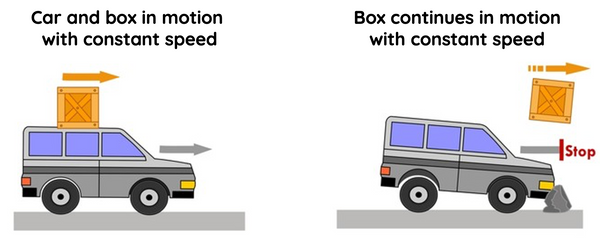# Newton's Laws of Motion

This topic is part of the HSC Physics course under the section Forces.

### HSC Physics Syllabus

• using Newton’s Laws of Motion, describe static and dynamic interactions between two or more objects and the changes that result from:

– a contact force
– a force mediated by fields
• investigate, describe and analyse the acceleration of a single object subjected to a constant net force and relate the motion of the object to Newton’s Second Law of Motion through the use of: (ACSPH062, ACSPH063)

– qualitative descriptions
– graphs and vectors
– deriving relationships from graphical representations including F=ma and relationships of uniformly accelerated motion

### Newton's Laws of Motion

As a HSC Physics student, understanding Newton's Laws of Motion is crucial for grasping the fundamental principles of classical mechanics. In this article, we will explore the three laws, their implications, and how they apply to everyday situations.

### Newton's First Law of Motion: The Law of Inertia

The first law of motion, also known as the Law of Inertia, states that an object at rest will stay at rest, and an object in motion will continue moving at a constant velocity, unless acted upon by a net external force.Inertia is present in both static and dynamic equilibrium states, when the net force equals zero in horizontal and vertical directions.

Example 1.1: Consider a car with a box on its roof travelling at constant speed. The box is not tied to the roof in any way. When the car comes to a sudden stop, the box will be flung forward at constant speed. The box will eventually come to a stop due to the effects of weight force and air resistance. If these forces are not present, then the box will continue to move at constant speed indefinitely.

Example 1.2: Imagine a book resting on a table. The book will remain at rest because there are no net external forces acting on it (static equilibrium). If you apply force by pushing the book, it will move. However, if you stop pushing, the book will eventually come to a halt due to the external force of friction.

### Newton's Second Law of Motion: The Law of Acceleration

The second law of motion describes how the velocity of an object changes when subjected to an external force. It states that the acceleration of an object is directly proportional to the net external force acting on it and inversely proportional to its mass.Mathematically, this can be expressed as:

$$F_{net} =m \times a$$

where F is the net force, m is the mass of the object, and a is its acceleration.

Example 2.1: A car with a mass of 1,000 kg accelerates from rest at a rate of 3 m/s².

To calculate the force needed, use the formula F = m × a:

$$F_{net} = 1000 \times 3 = 3000 N$$

Therefore, a force of 3,000 N in the positive direction must be applied to the car to achieve the desired acceleration.

### Newton's Third Law of Motion: Action and Reaction

The third law of motion states that for every action, there is an equal and opposite reaction. In other words, when an object exerts a force on a second object, the second object exerts an equal and opposite force on the first object.Example 3.1: When you push a box across the ground, you exert a horizontal force on the box. In response, the box exerts an equal and opposite horizontal force on you, causing you to slide backwards.

Example 3.2: When you jump off a diving board, you exert a downward force on the board. In response, the board exerts an equal and opposite upward force on you, propelling you into the air.